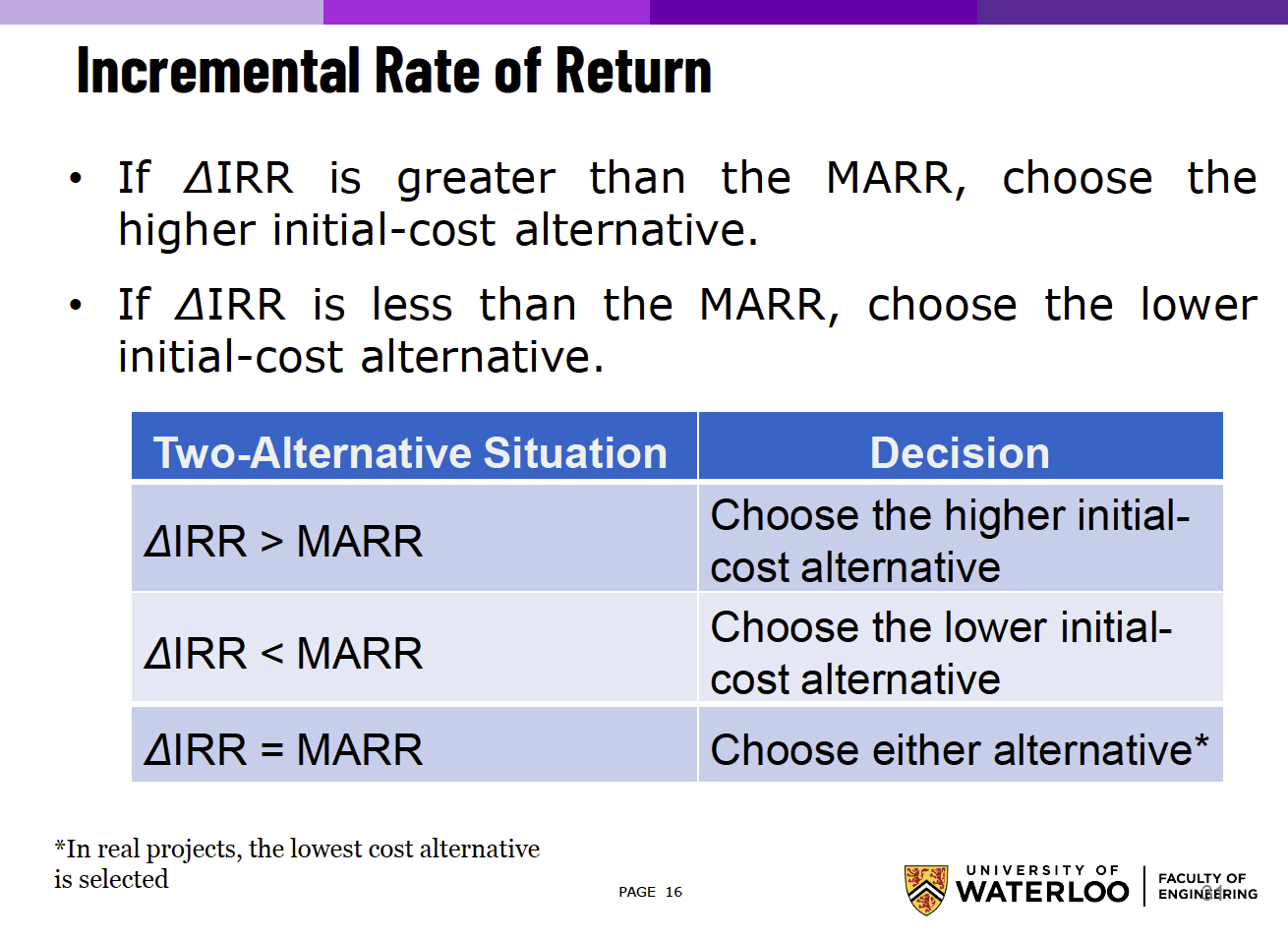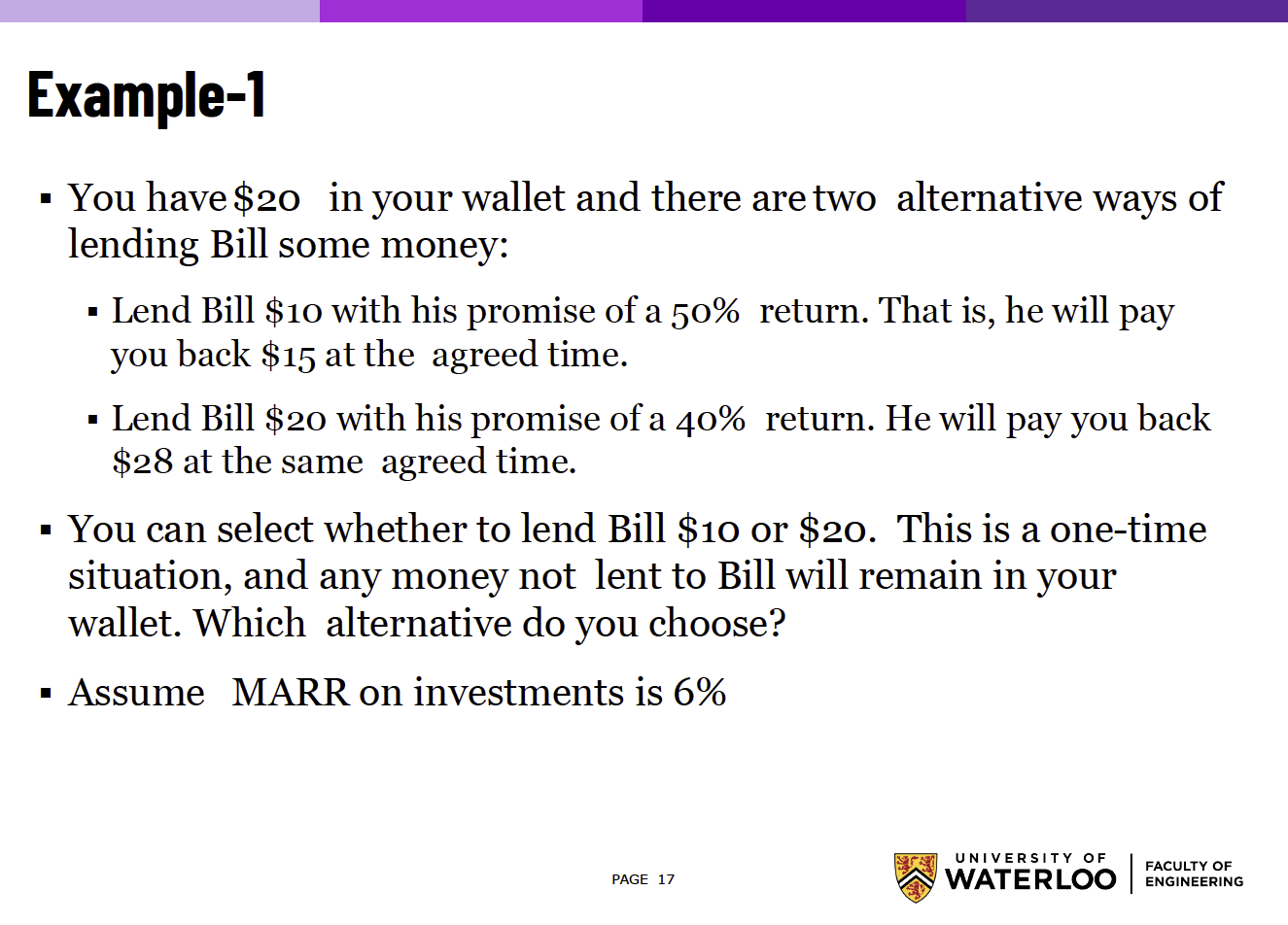# Worth Analysis §

Learned in ECE192.

### Present Worth Analysis §

This is just using the Present Worth Factor.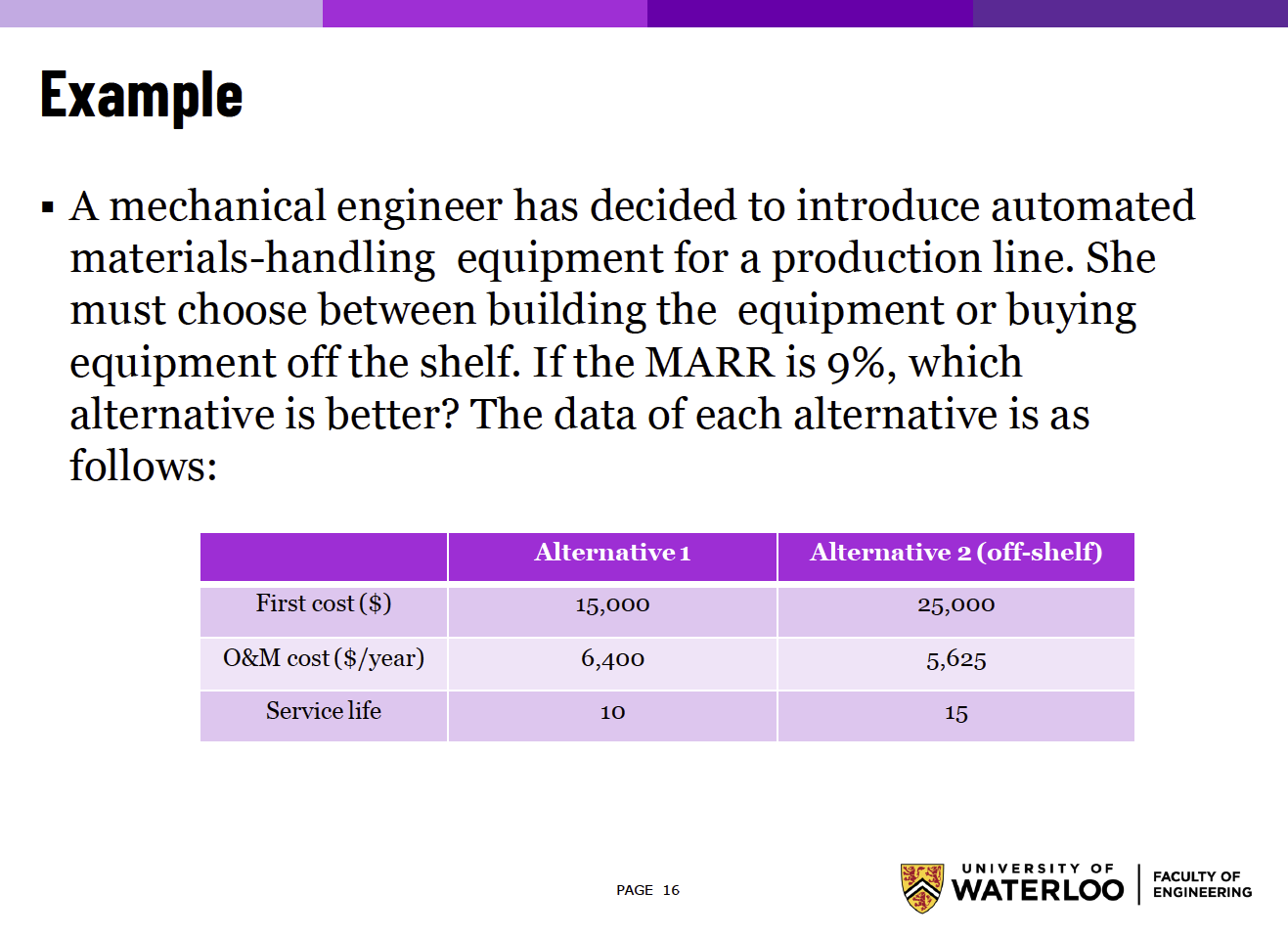You need to be very careful because both alternatives have different service lives (pages 17-19 show the wrong calculations). We need to apply the repeated lives method to get a fair comparison.

Just visualize the following cash flow diagram: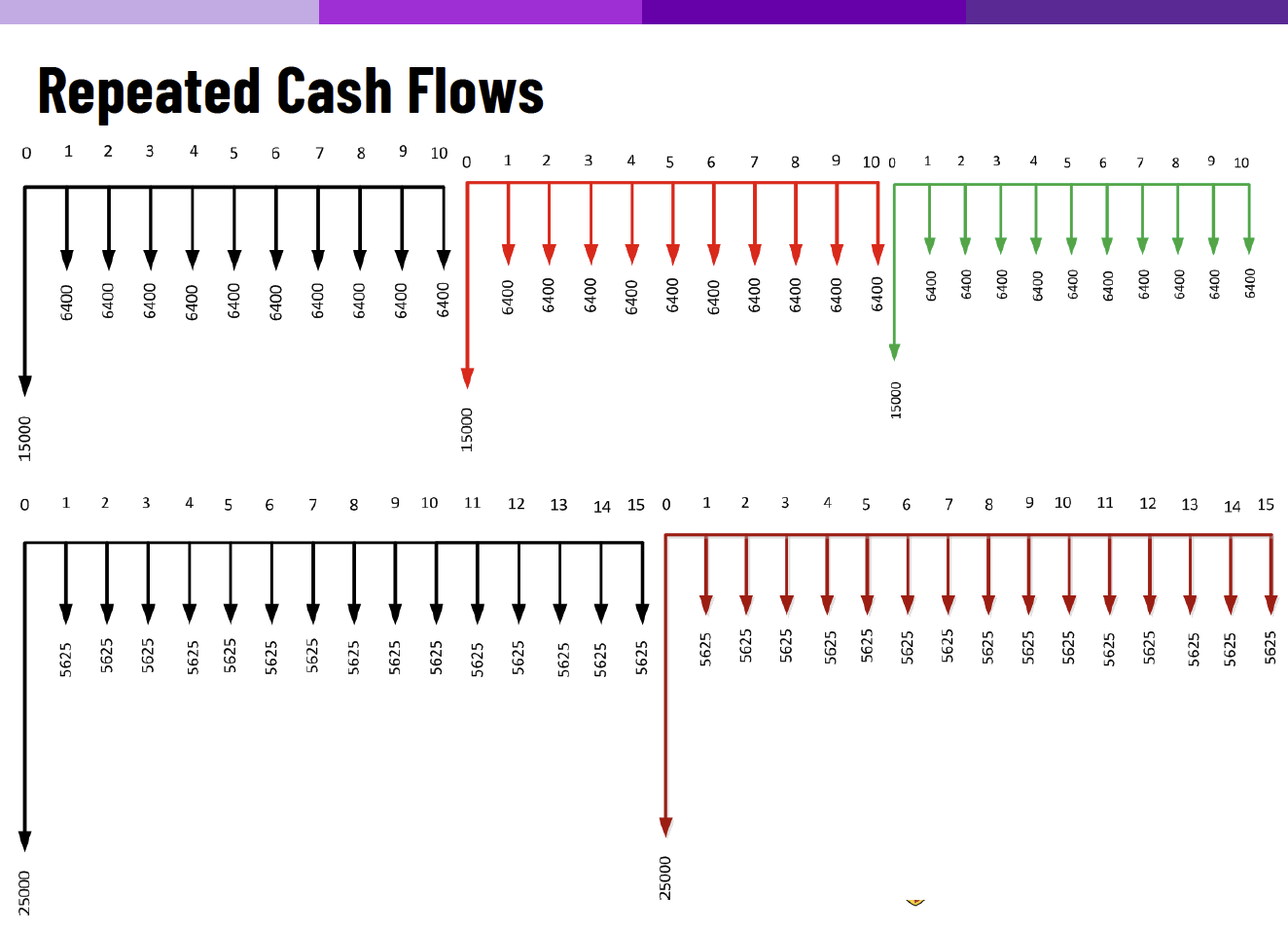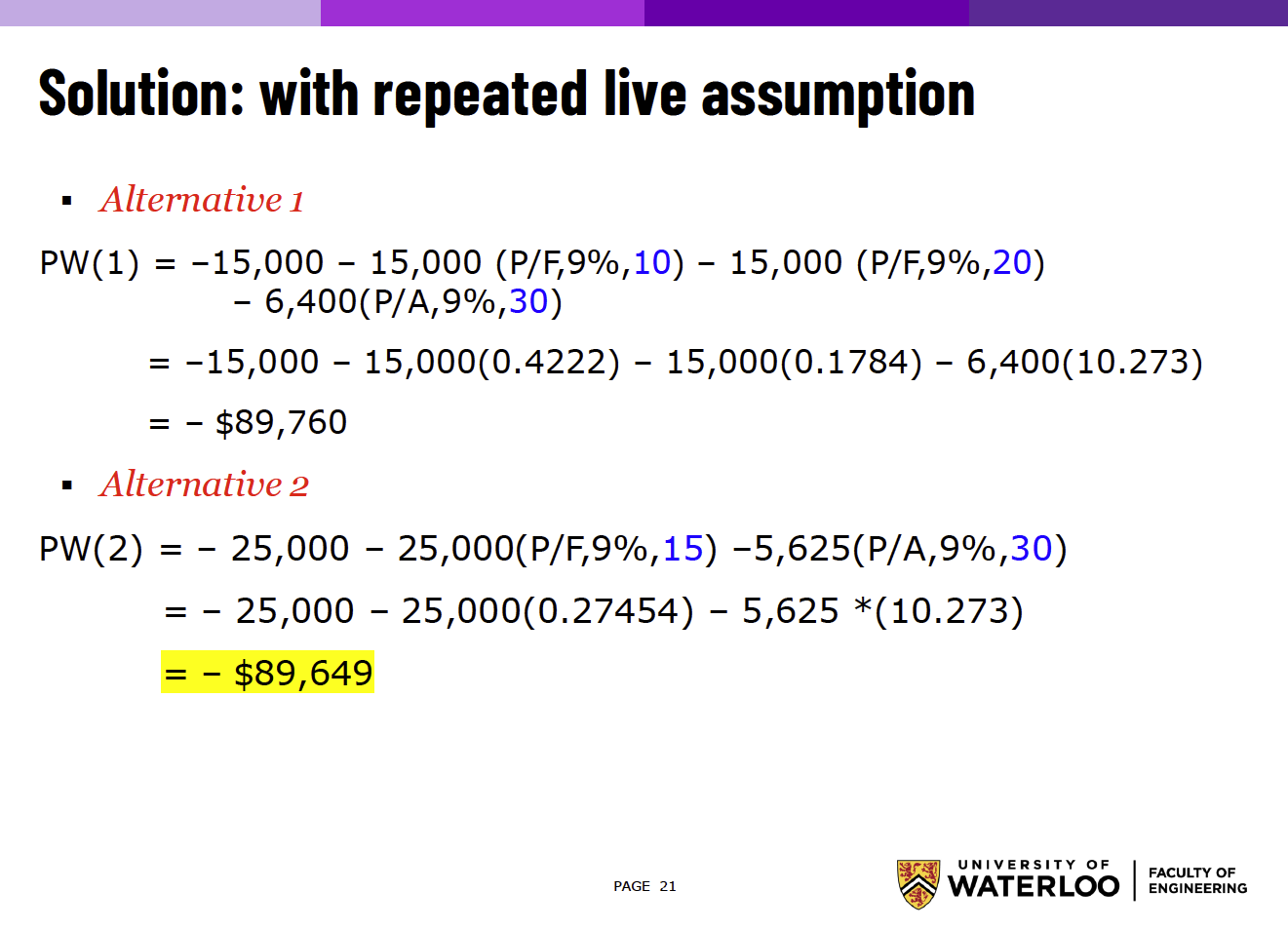### Future Worth Analysis §

This is just using the Compound Amount Factor.

Example is super similar, just need to be careful to wrap your head around the calculation.

• Everything is relative to the future, so you don’t need to worry about calculating in terms of the present. This was confusing to me for the \$15,000 cost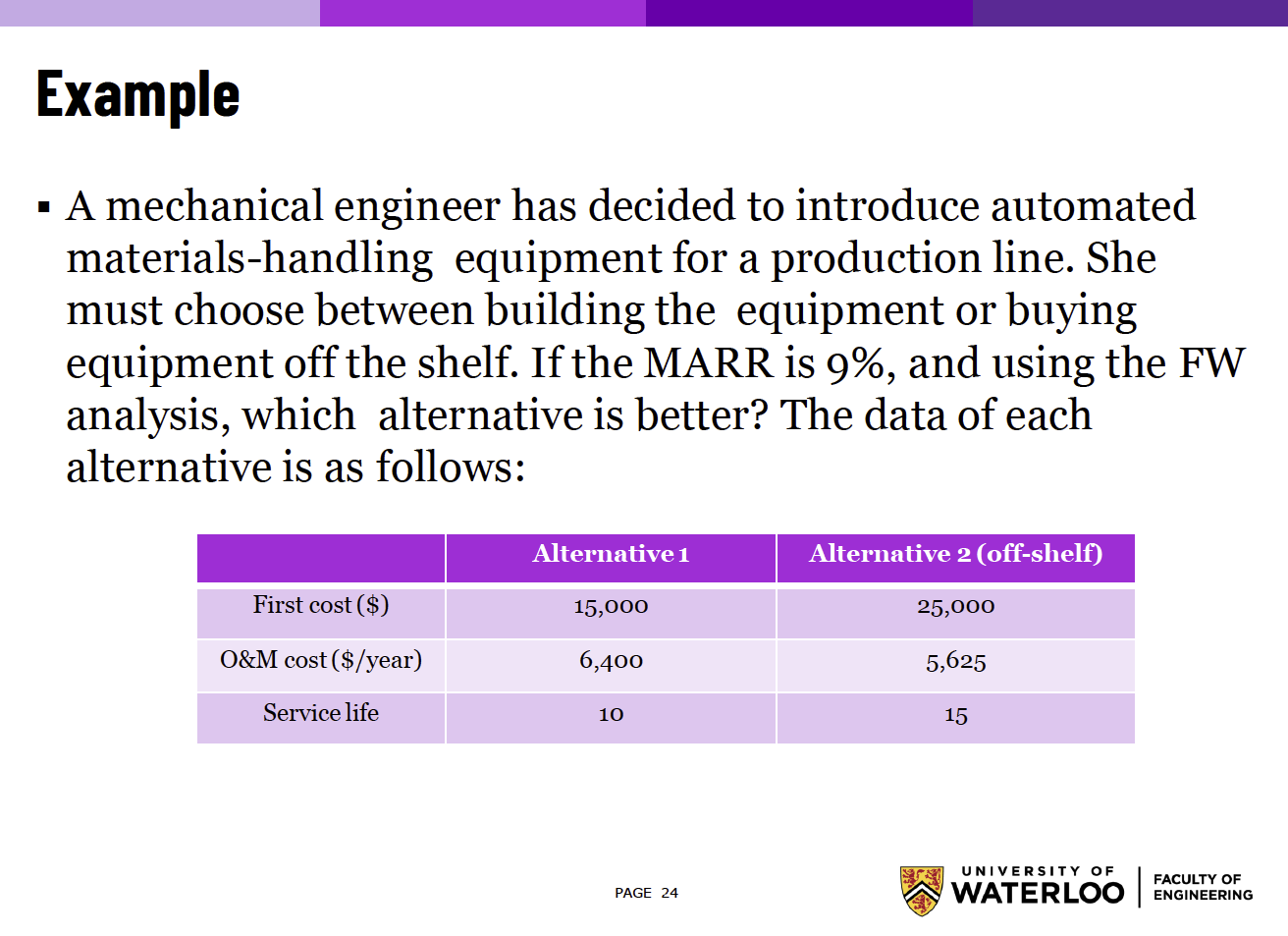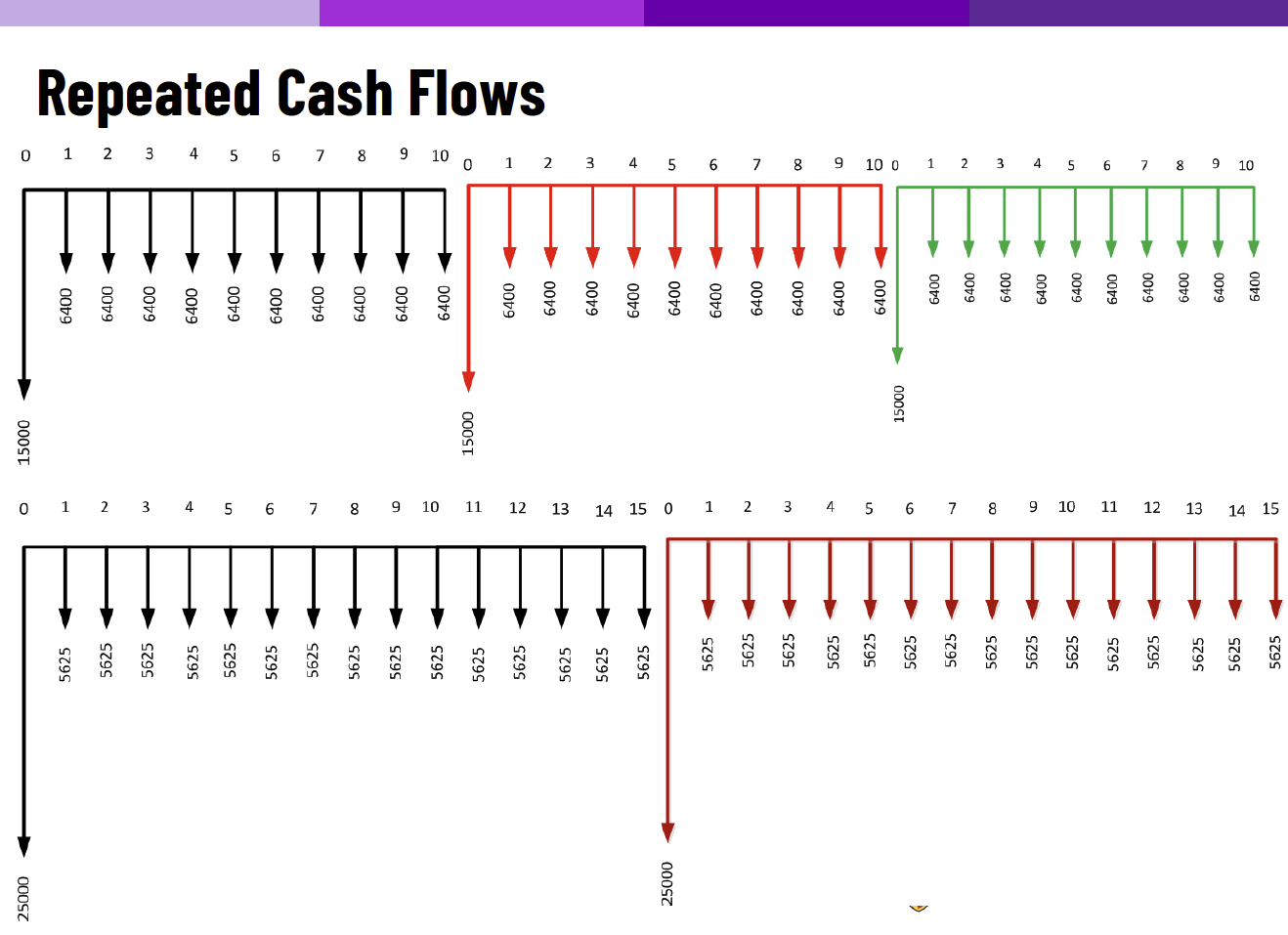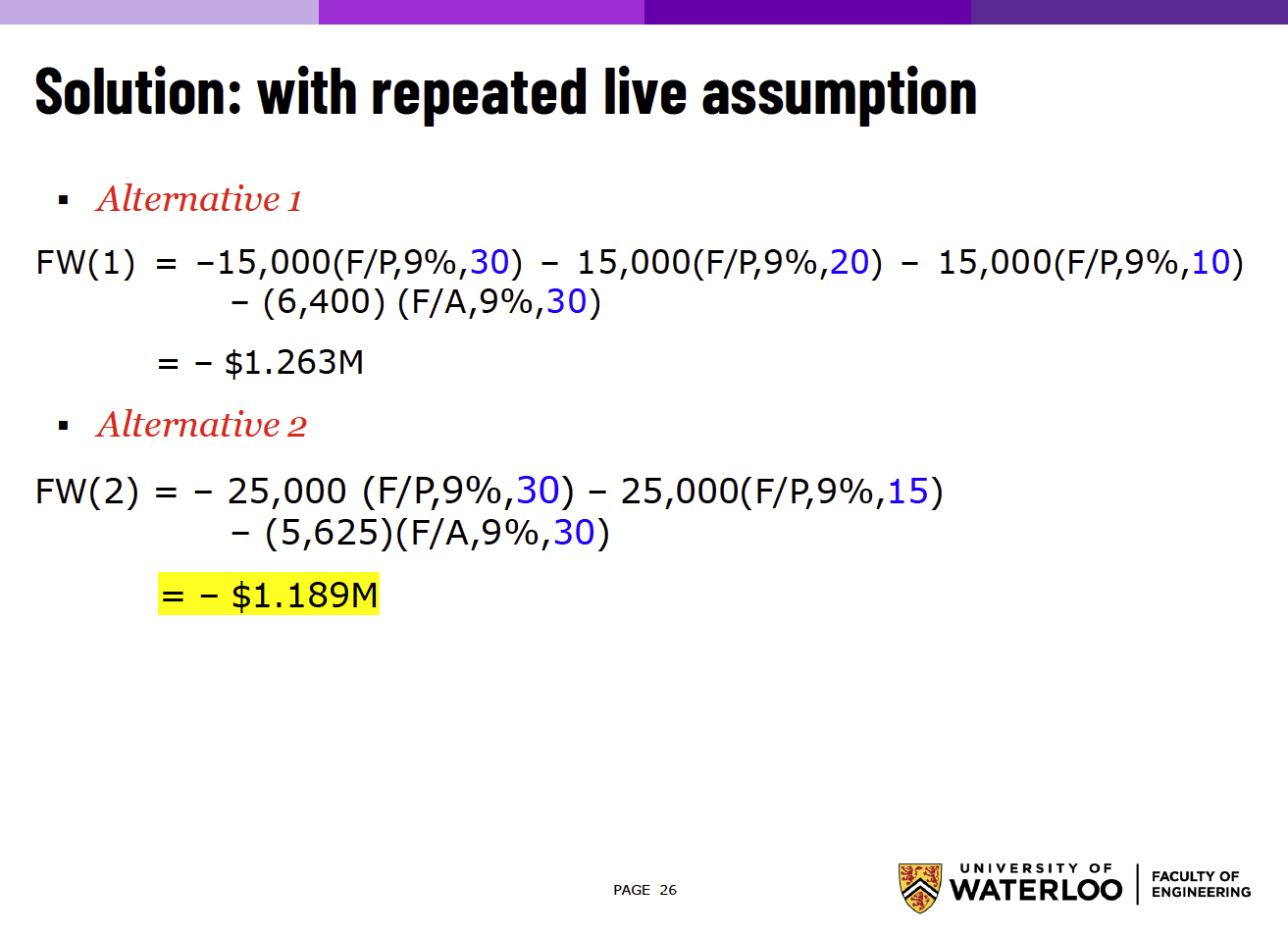#### Capitalized Cost Analysis §

This is so easy. It’s basically the Series Present Worth Factor where .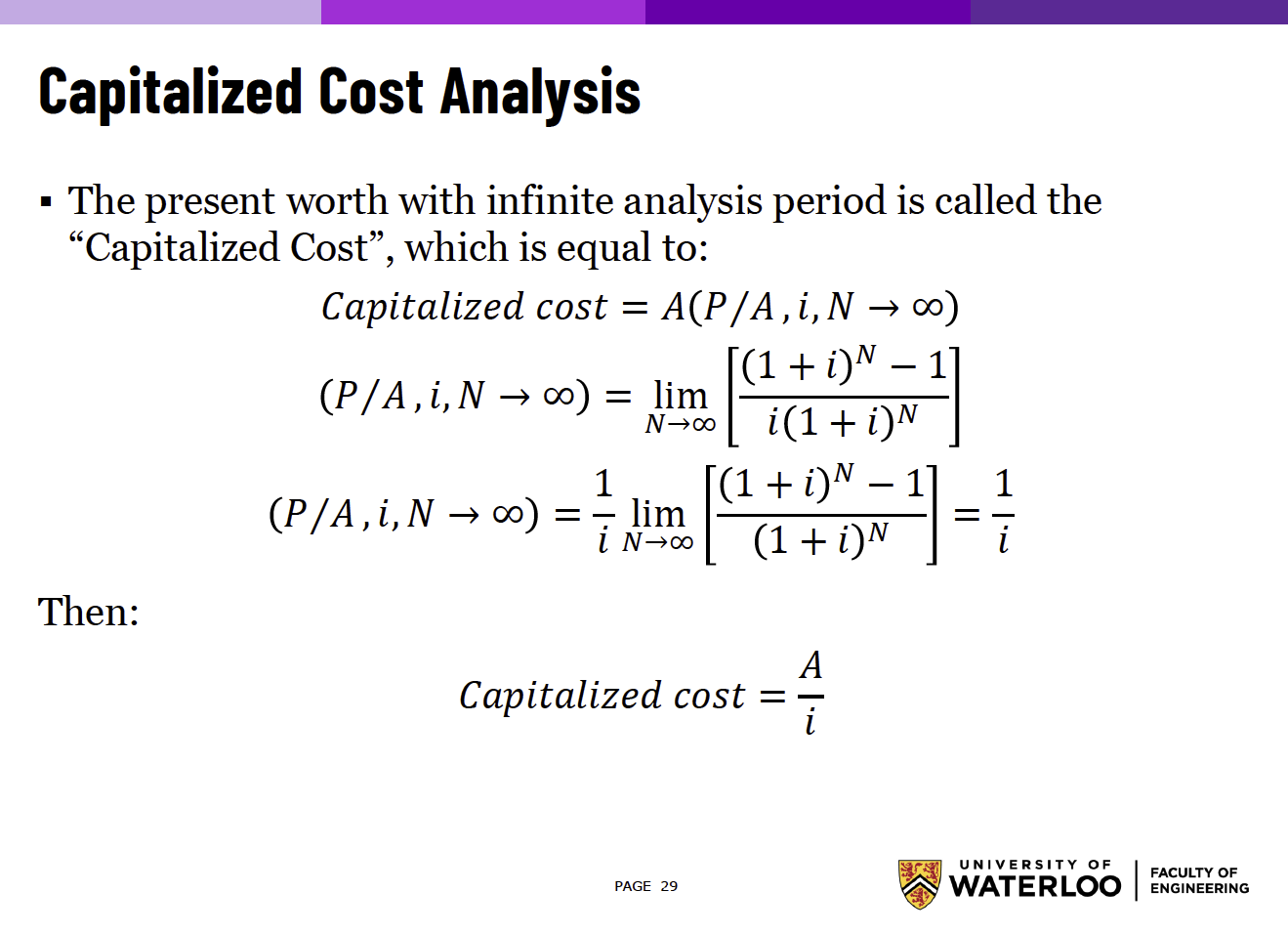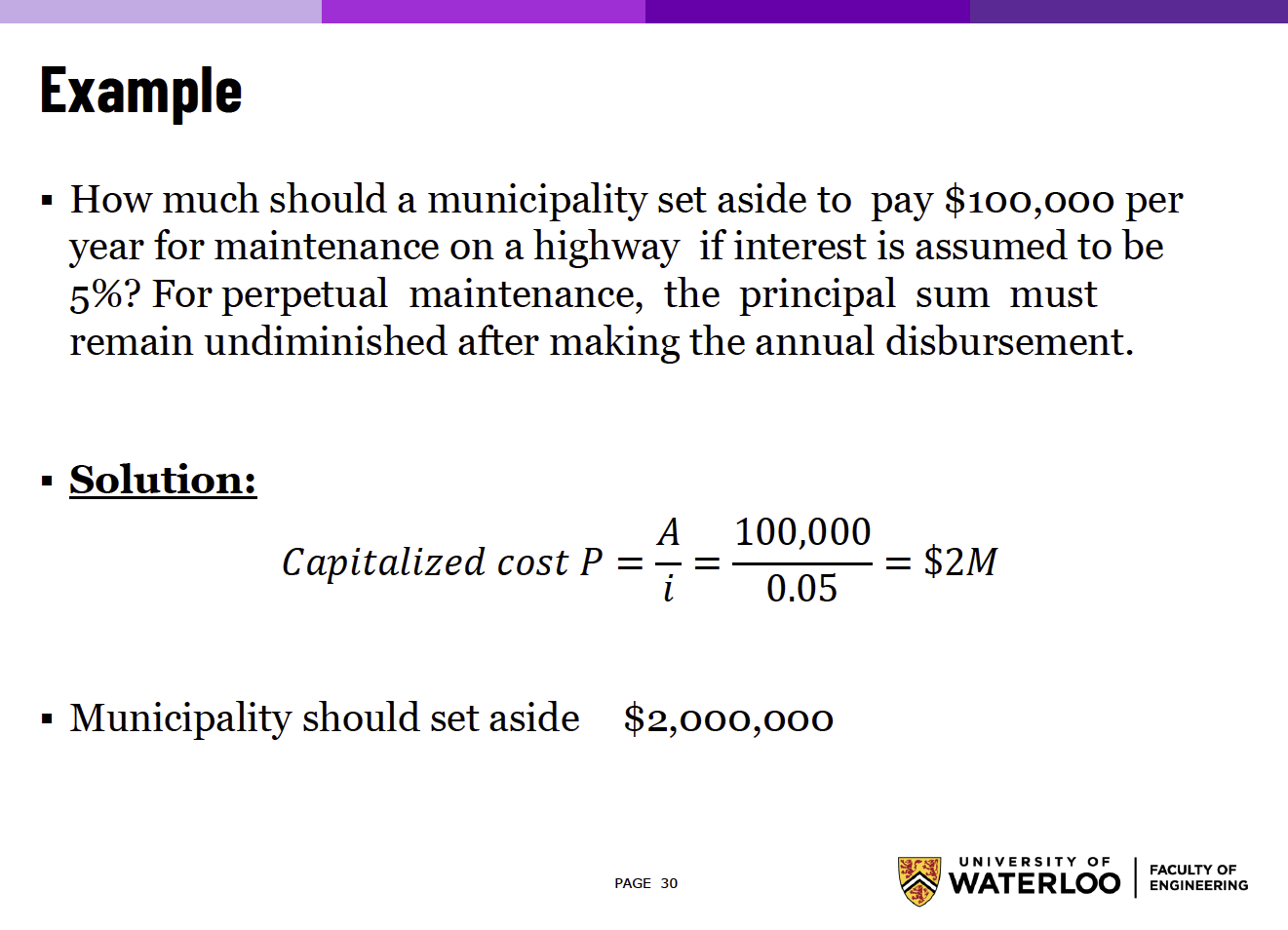### Annual Worth Analysis §

The AW, or Equivalent Uniform Annual Worth (EUAW), represents a measure of project profitability as an equivalent annual value.

This is basically using Capital Recovery Factor.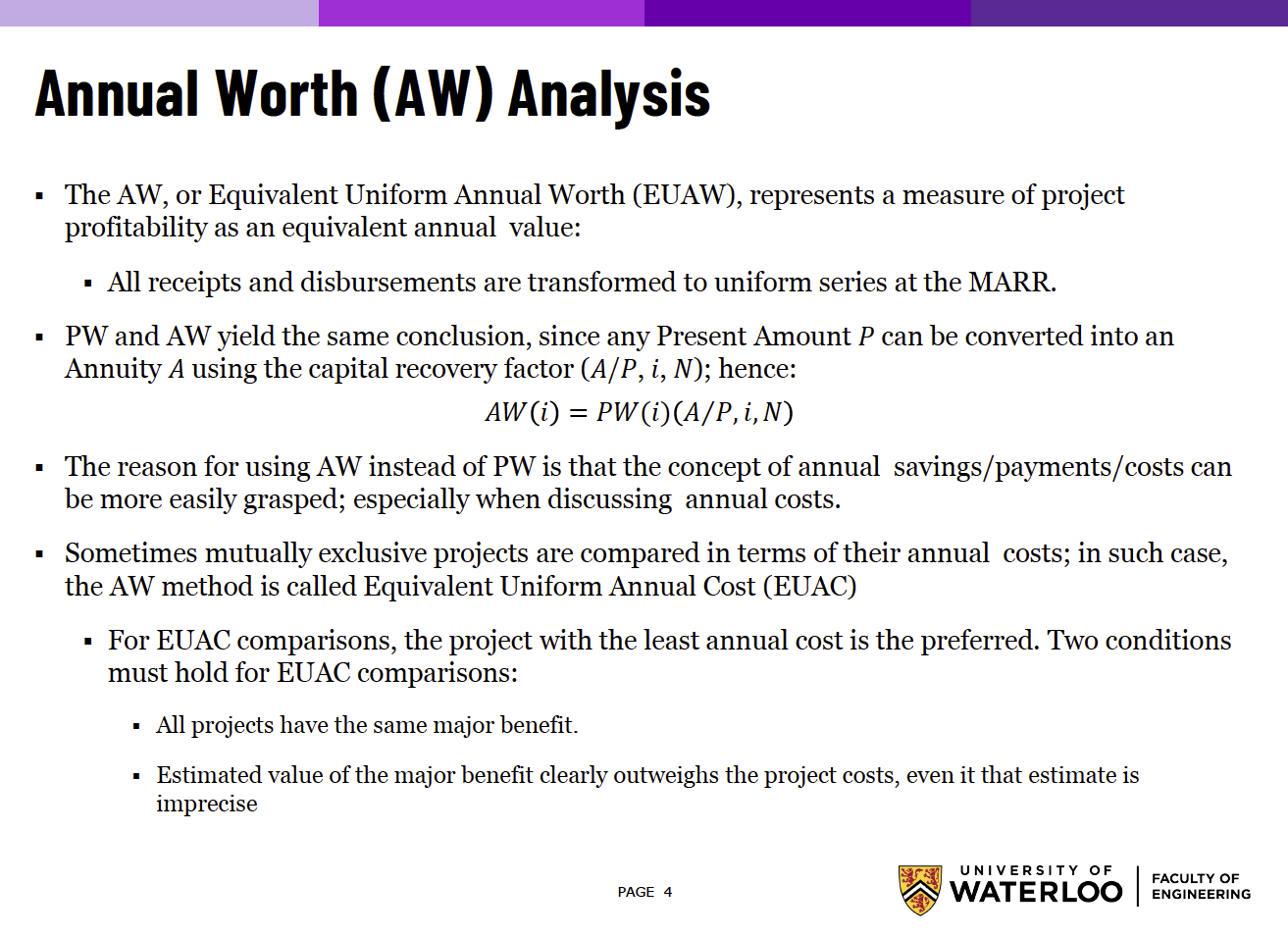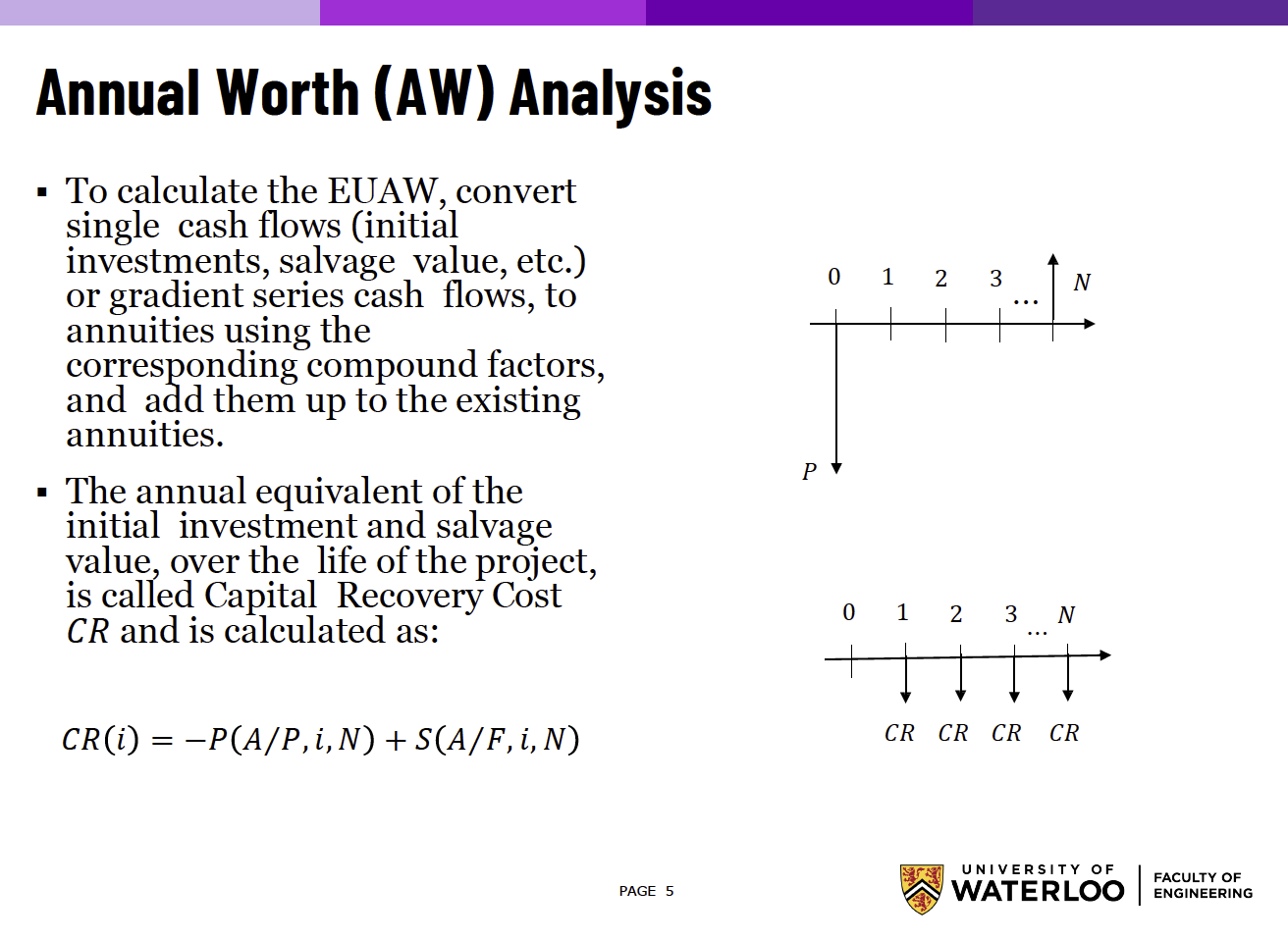Example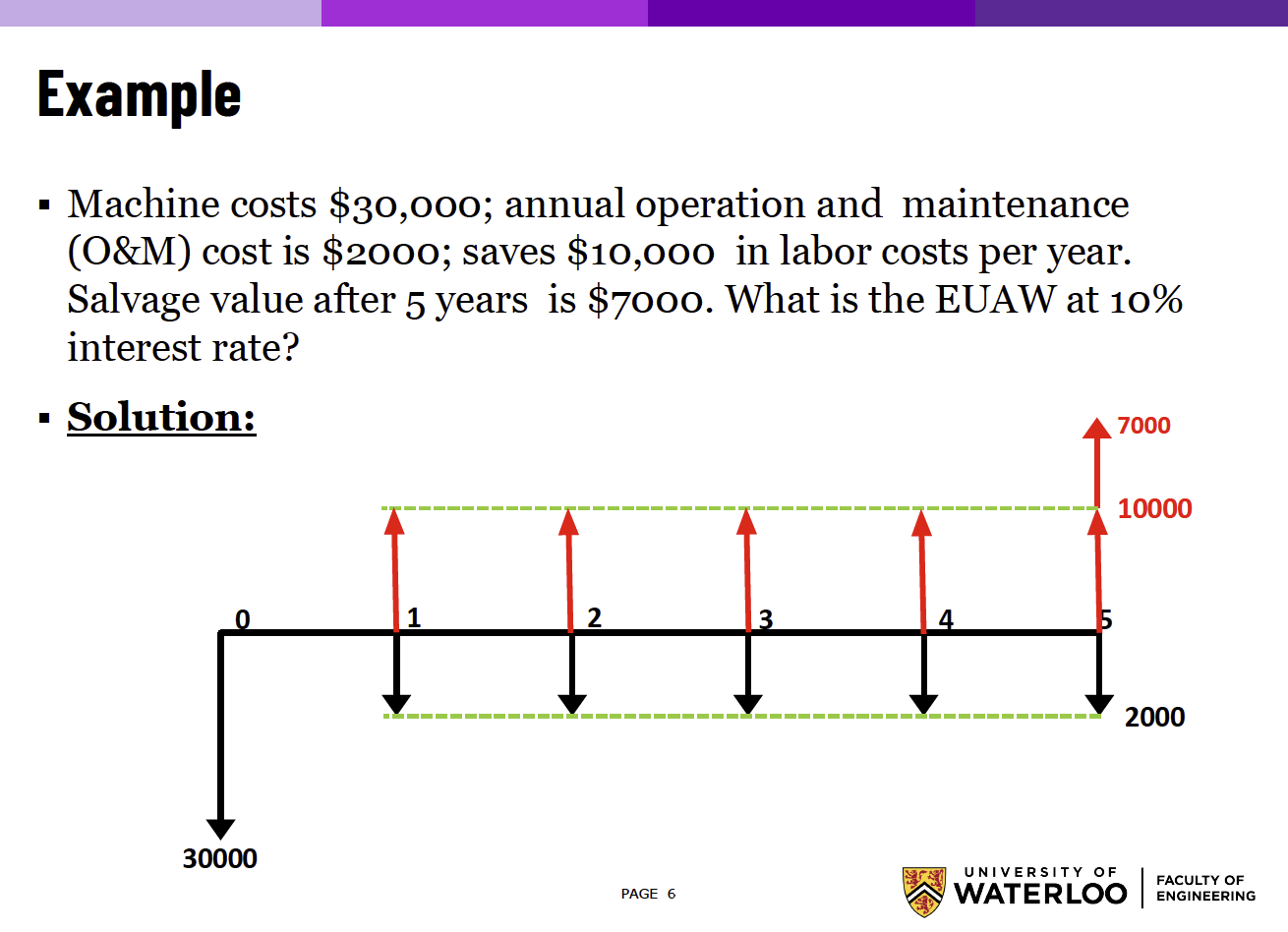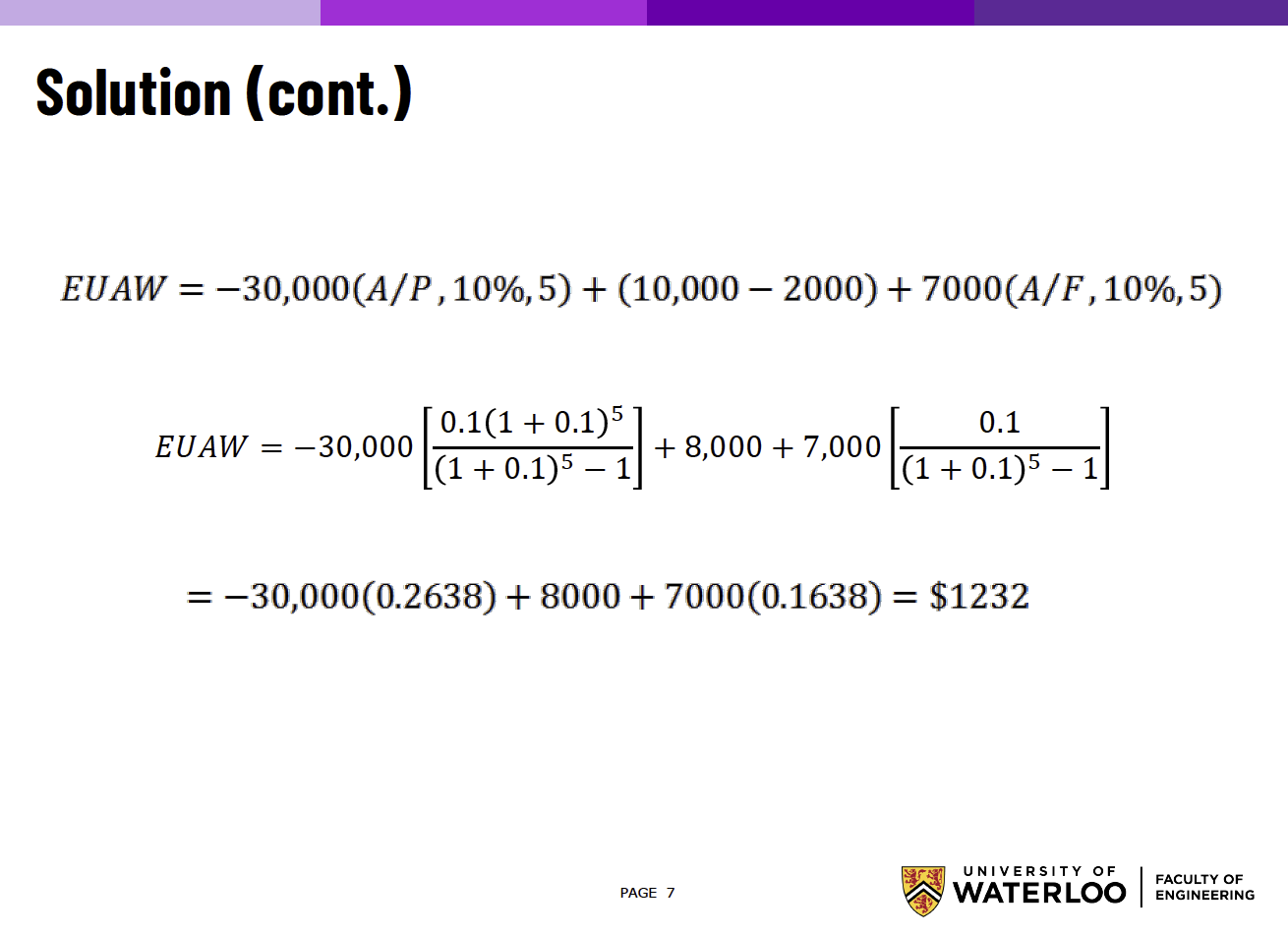Another example: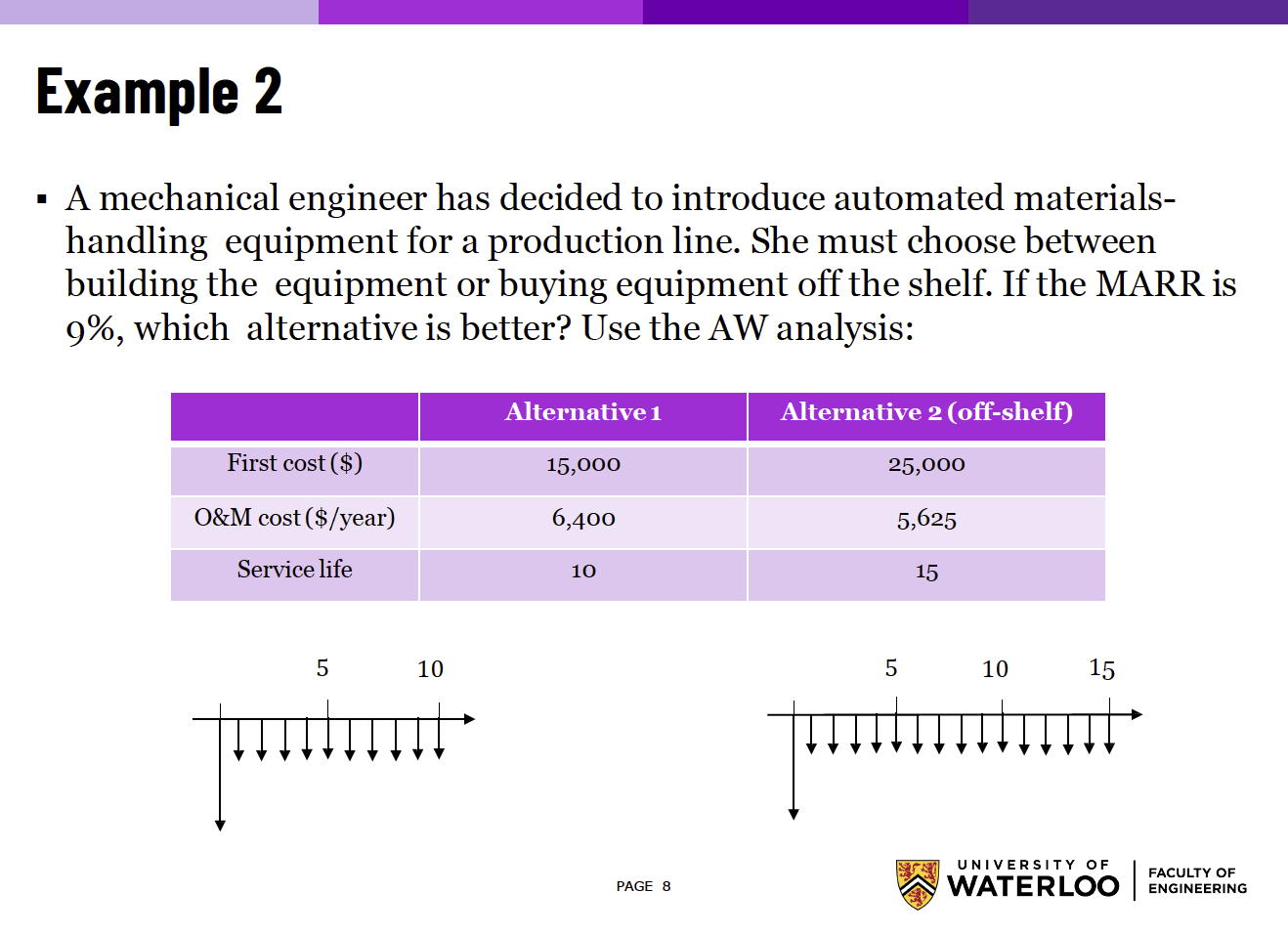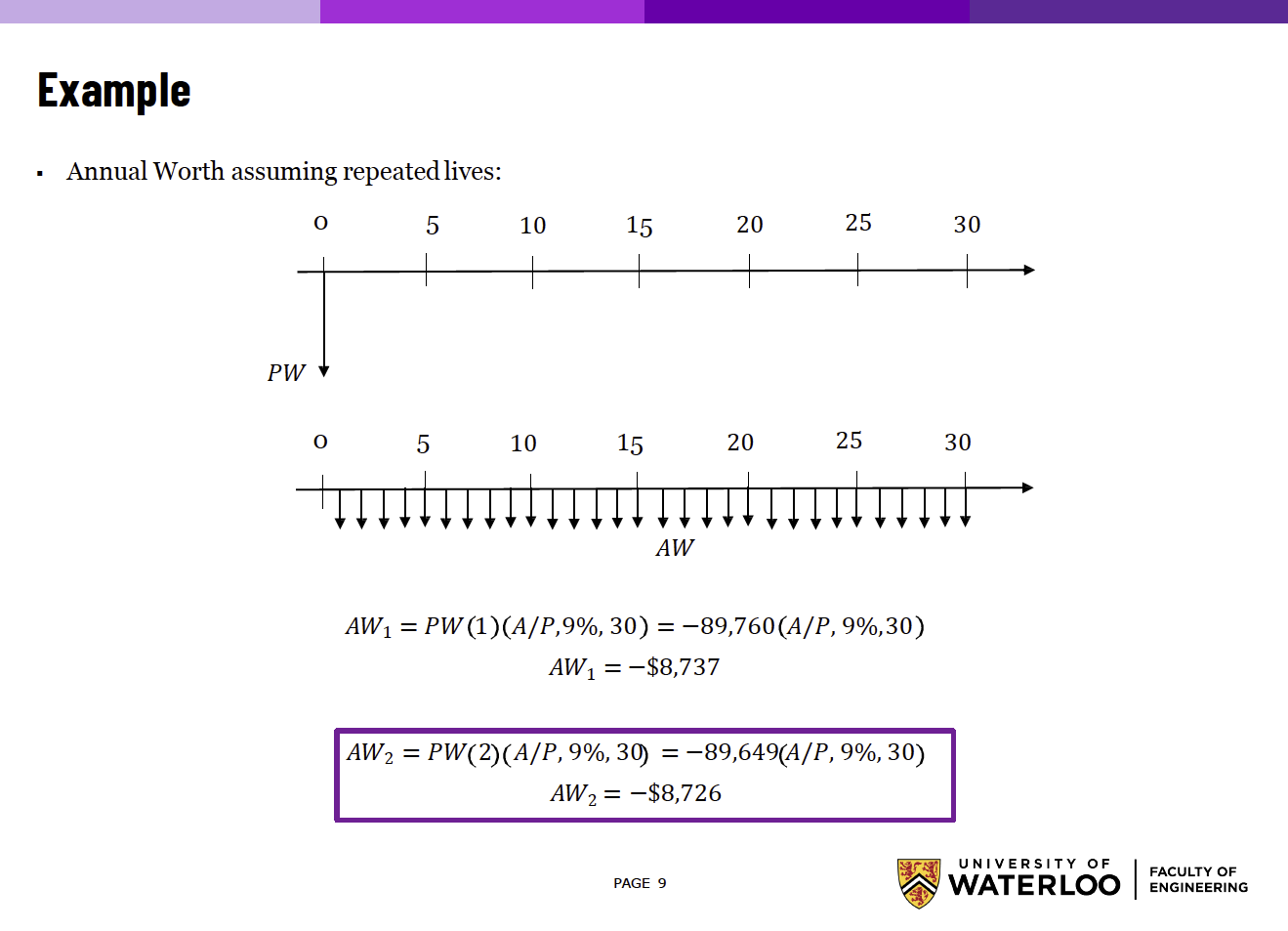### Rate of Return (ROR) Analysis §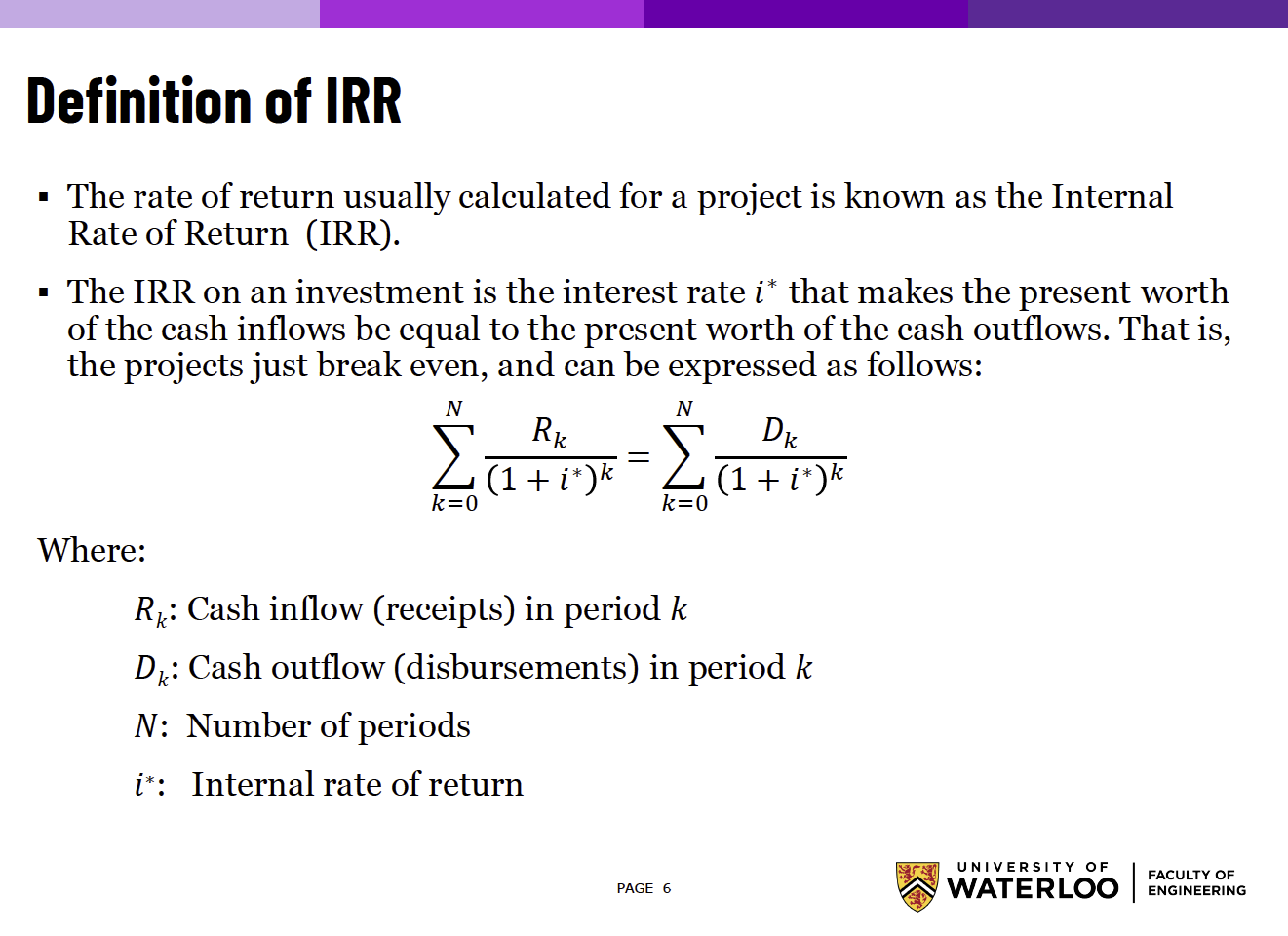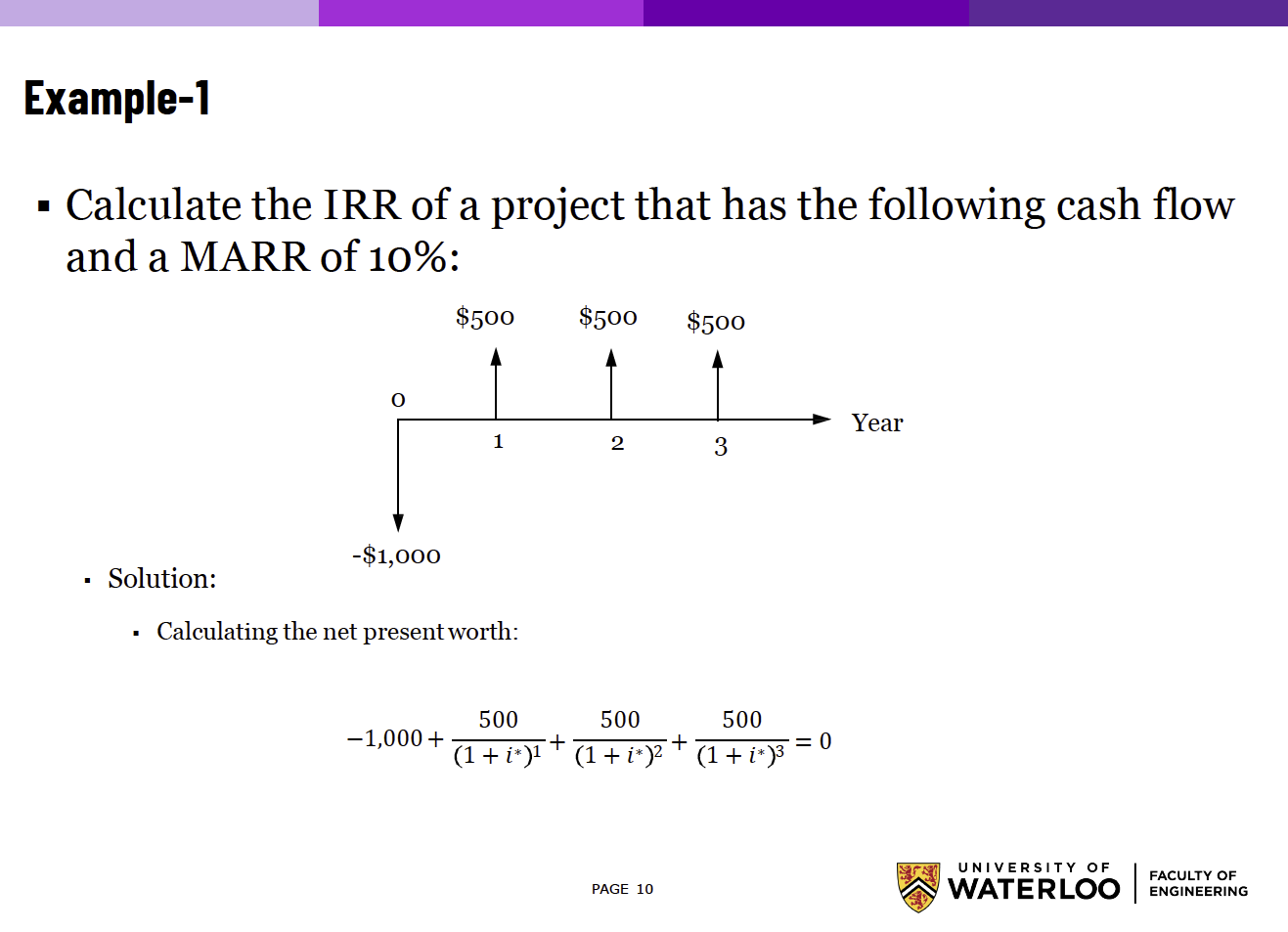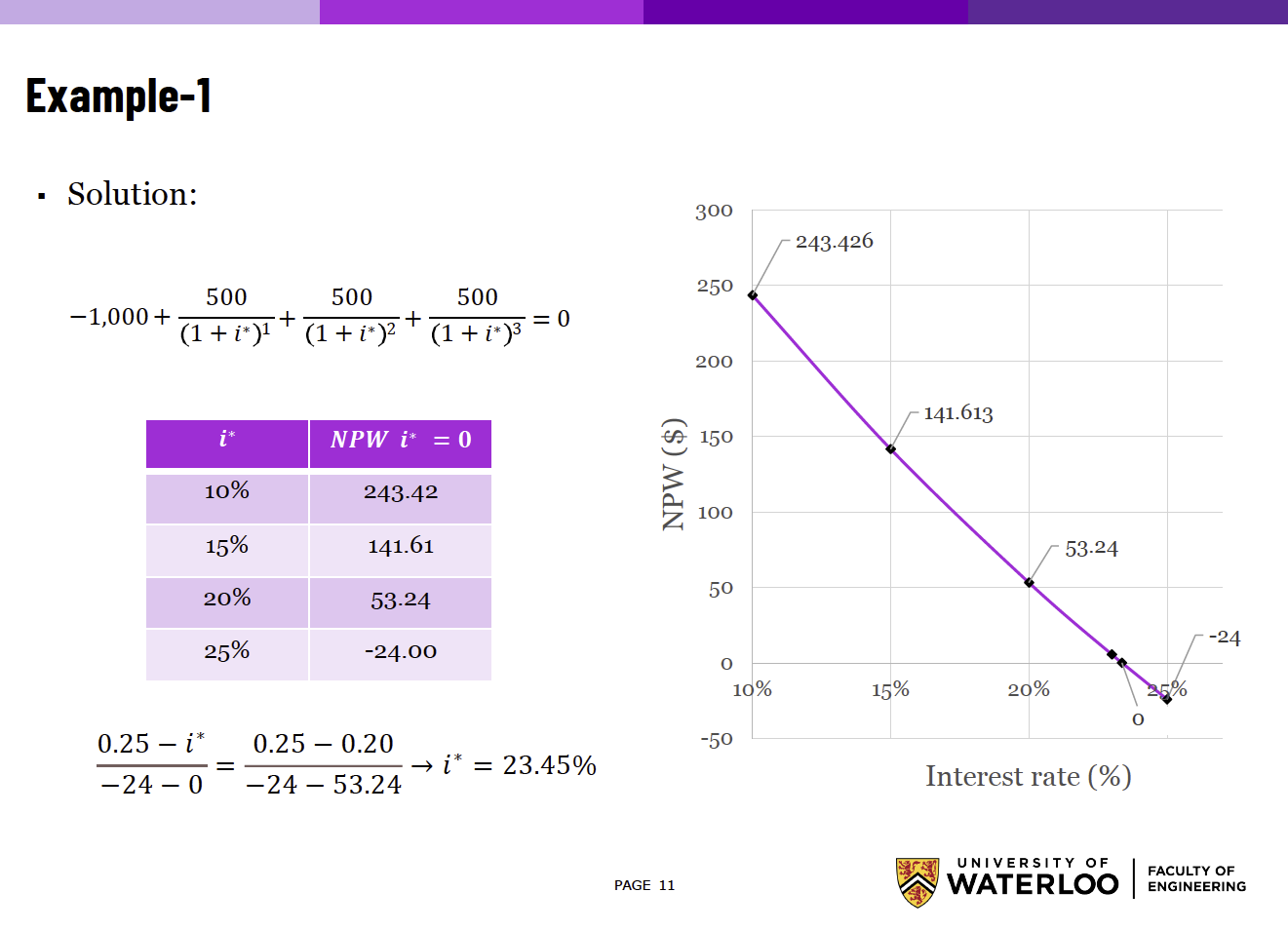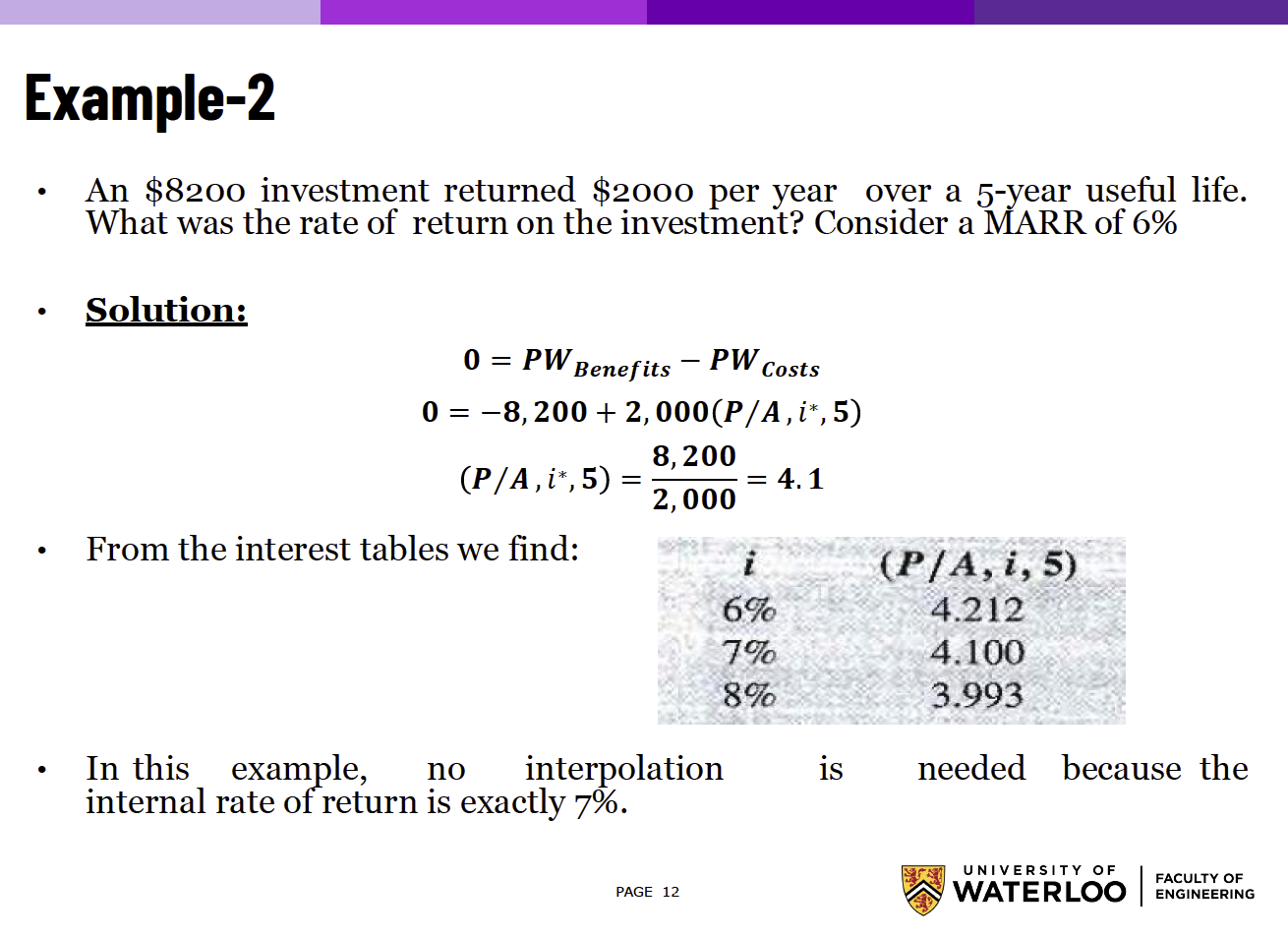#### Incremental Rate of Return §